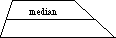index: click on a letter A B C D E F G H I J K L M N O P Q R S T U V W X Y Z A to Z index index: subject areas numbers & symbols sets, logic, proofs geometry algebra trigonometry advanced algebra & pre-calculus calculus advanced topics probability & statistics real world applications multimedia entrieswww.mathwords.com about mathwords website feedback

 Median of a Trapezoid A line segment connecting the midpoints of the legs of a trapezoid. The median is parallel to the bases. Note: Its length equals the arithmetic mean of the lengths of the bases.See also